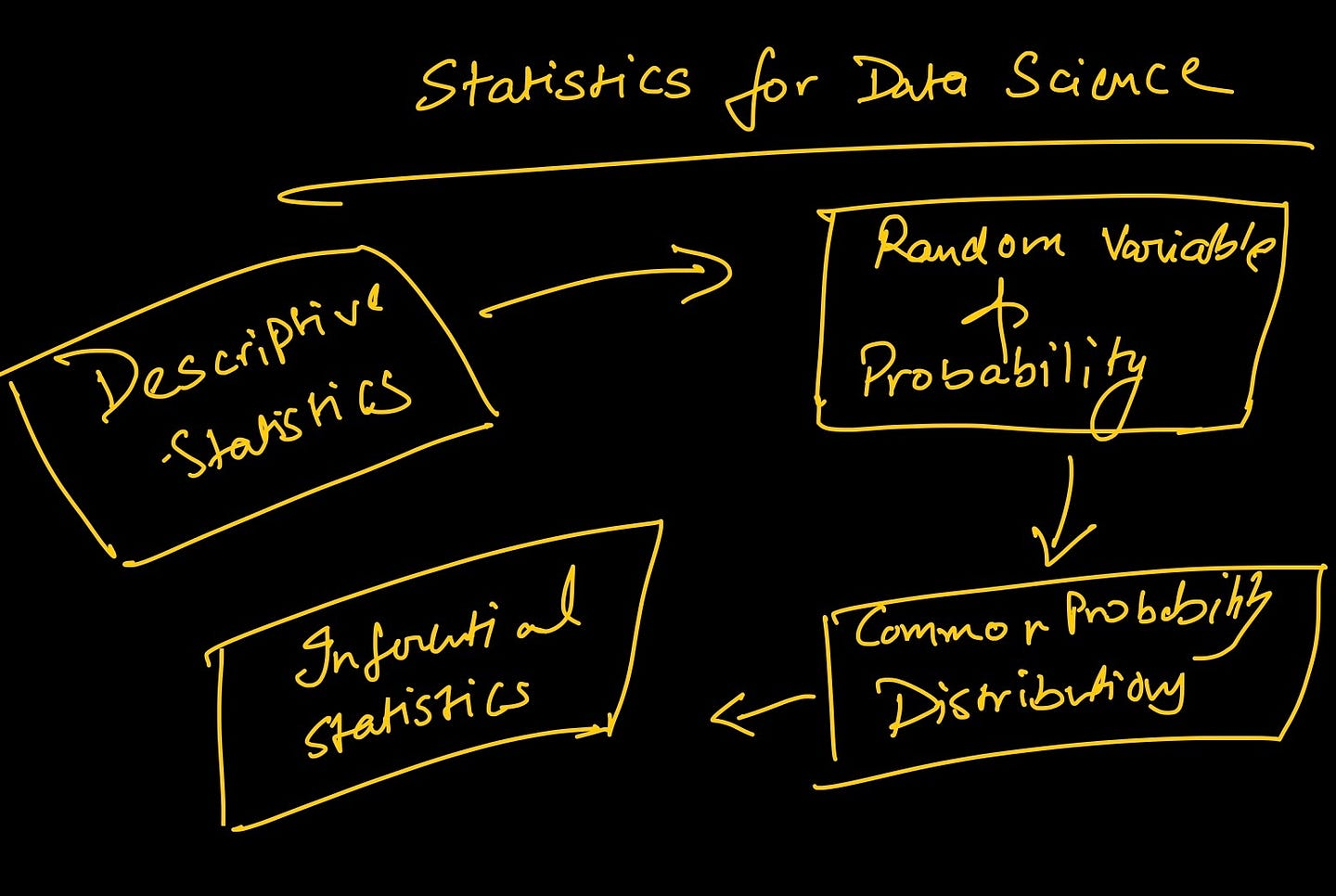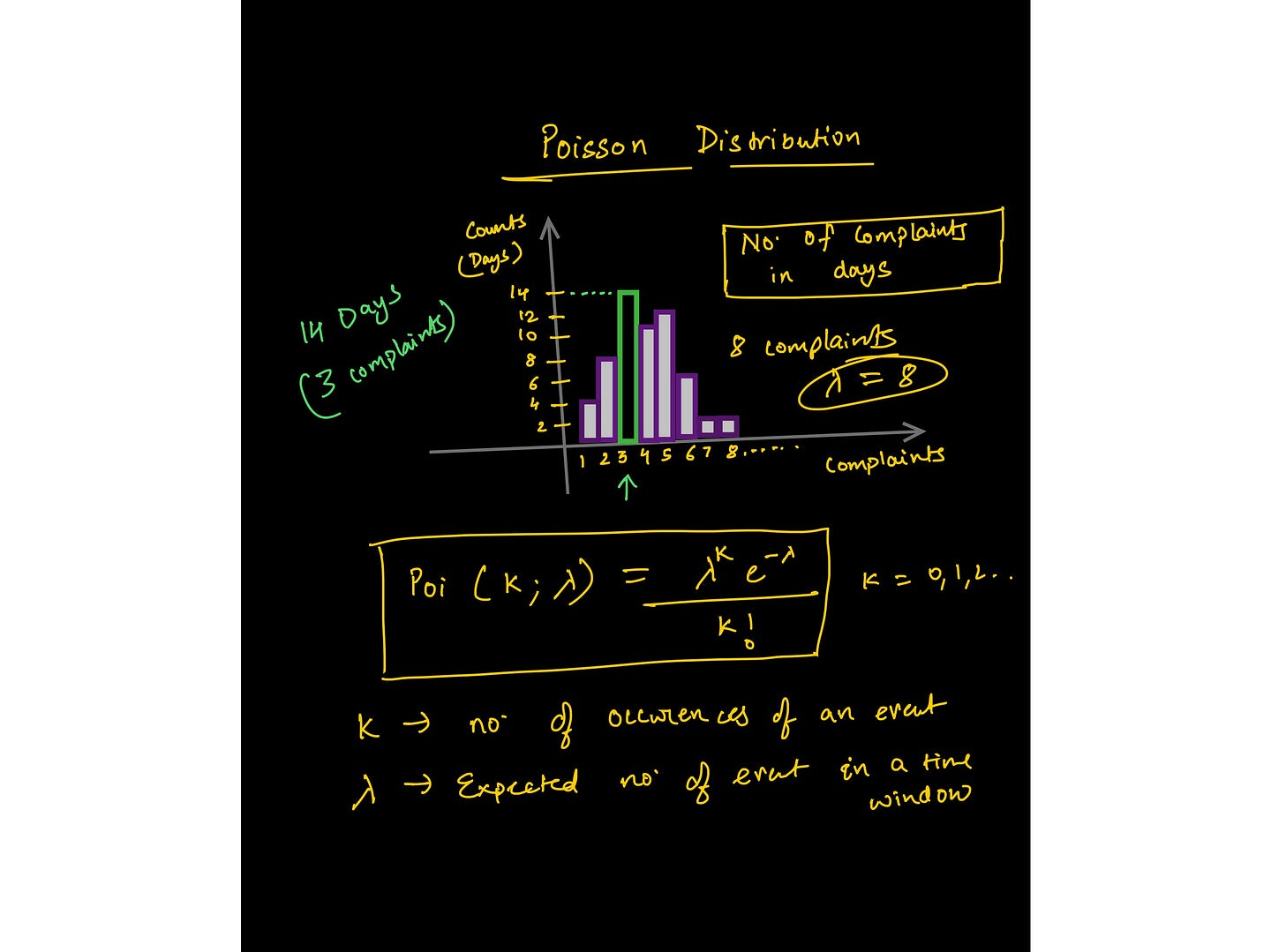Whether you decide to take Andrew Ng’s Machine Learning and Deep Learning course on YouTube or any Data Science bootcamp, you will need a certain degree of mathematical and statistical knowledge.

This will not only help you understand basic ML/DS concepts, but it will also help you make a long-lasting, robust career as a data professional.

This is a short and precise guide for all self-taught devs and beginners in the field of Data Science and Machine Learning.

There's a common question that pops up in all my training programs, LinkedIn courses, videos on YouTube, or newsletters. It's that when people start learning DS/ML, after a certain point they feel lost in mathematics or statistics and sometimes programming.

And I have always recommended learning or refreshing some mathematical concepts that underpin ML as it helps you build intuition which keeps you curious throughout your learning journey.

To back up this claim, here are the prerequisites and prework Google recommends before taking their Machine Learning Crash Course:

I’d recommend that you go through this article first and then look up all the links one by one and use this blog as a reference.

After going through the complete list of concepts and skills that are mentioned in the Google article, I also went through several books (Deep Learning by Ian Goodfellow, Deep Learning with Python by Francois Chollet, and several others).

From them, I tried to distill the essentials into three branches that you'll need to build a solid foundation for a career as a Data Analyst/Scientist/ML Engineer.

Following are the three pillars along with the a list of concepts that make for a good starter program:

## Programming for Complete Beginners in Data Science and Machine Learning

Programming means telling a computer predefined rules that help it process input data and then get the results.

Machine learning, on the other hand, is giving the machine the results and data to find the rules that best approximate the relationship between the data and the results.

Programming offers that base platform which you can use to automate, verify, and solve problems of any scale.

The next question is which language should you learn?

Since most of the courses, libraries, and books are written to support Python infrastructure, I recommend learning Python and so does Google’s guide. Which language you use is a personal choice and a lot of it depends on the type of problem you’re trying to solve.

Most beginners prefer Python as it is the best way to develop end-to-end projects and there is a very large community of fellow developers who can help you. Chances are that ~90% of the problems that you’ll encounter in your journey (especially in the beginning phase) are already solved and documented for you.

## 1. Essential Python Programming for Machine Learning

Most data roles are programming-based except for a few like business intelligence, market analysis, and product analysis.

I am going to focus on technical data jobs that require expertise in at least one programming language. I personally prefer Python over any other language because of its versatility and ease of learning  – hands-down a good pick for developing end-to-end projects.

Here are some of the topics/libraries you should study for data science/ML:

• Common data structures (data types, lists, dictionaries, sets, tuples), writing functions, logic, control flow, searching and sorting algorithms, object-oriented programming, and working with external libraries.
• Writing Python scripts to extract, format, and store data into files or back to databases.
• Handling multi-dimensional arrays, indexing, slicing, transposing, broadcasting and pseudorandom number generation using NumPy.
• Performing vectorized operations using scientific computing libraries like NumPy.
• Manipulate data with Pandas  –  series, dataframe, indexing in a dataframe, comparison operators, merging dataframes, mapping, and applying functions.
• Wrangling data using pandas  –  checking for null values, imputing it, grouping data, describing it, performing exploratory analysis, and so on.
• Data Visualization using Matplotlib  – the API hierarchy, adding styles, color, and markers to a plot, knowledge of various plots and when to use them, line plots, bar plots, scatter plots, histograms, boxplots, and seaborn for more advanced plotting.

## 2. Essential Mathematics for Data Science and Machine Learning

There are practical reasons why Math is essential for folks who want a career as an ML practitioner, Data Scientist, or Deep Learning Engineer.

### Use linear algebra to represent dataAn image from the course: https://www.wiplane.com/p/foundations-for-data-science-ml

ML is inherently data-driven – data is at the heart of machine learning. We can think of data as vectors , an object that adheres to arithmetic rules. This leads us to understand how rules of linear algebra operate over arrays of data.

### Use calculus to train ML models

Model training happens does not happen “automatically”. Calculus is what drives the learning of most ML and DL algorithms.

One of the most commonly used optimization algorithms  ( gradient descent)  is an application of partial derivatives.

A model is a mathematical representation of certain beliefs and assumptions. It is said to learn (approximate) the process (linear, polynomial, etc) how the data is provided, was generated in the first place, and then make predictions based on that learned process.

Important topics include:

• Basic algebra variables, coefficients, equations, functions — linear, exponential, logarithmic, etc.
• Linear Algebra   scalars, vectors, tensors, Norms(L1 & L2), dot product, types of matrices, linear transformation, representing linear equations in matrix notation, solving linear regression problem using vectors and matrices.
• Calculus derivatives and limits, derivative rules, chain rule (for backpropagation algorithm), partial derivatives (to compute gradients), the convexity of functions, local/global minima, the math behind a regression model, applied math for training a model from scratch.

## 3. Essential Statistics for Data Science

Every organisation today is striving to become data-driven. To achieve that, Analysts and Scientists need to be able to use data in different ways in order to drive decision making.

### Describing data — from data to insights

Data always comes in raw and ugly. The initial exploration tells you what’s missing, how the data is distributed, and what’s the best way to clean it to meet the end goal.

In order to answer the questions you've defined, descriptive statistics enables you to transform each observation in your data into insights that make sense.

### Quantifying uncertainty

Furthermore, the ability to quantify uncertainty is the most valuable skill that is highly regarded at any data company. Knowing the chances of success in any experiment/decision is crucial for all businesses.

Here are a few of the main staples of statistics that constitute the bare minimum:Image from the lecture on Poisson distribution — https://www.wiplane.com/p/foundations-for-data-science-ml
• Estimates of location  – mean, median and other variants of these.
• Estimates of variability
• Correlation and covariance
• Random variables  – discrete and continuous
• Data distributions –  PMF, PDF, CDF
• Conditional probability –  bayesian statistics
• Commonly used statistical distributions –  Gaussian, Binomial, Poisson, Exponential.
• Important theorems  – Law of large numbers and Central limit theorem.Image from the lecture on Poisson distribution — https://www.wiplane.com/p/foundations-for-data-science-ml

Every beginner-level data science enthusiast should focus on these three pillars before diving into any core data science or core ML course

## How to Learn these Foundational DS and ML Concepts

I created a learning roadmap that you can find here. It also told you what to learn and was also loaded with resources, courses, and programs that you can check out.

But there are a few inconsistencies in the recommended resources and the roadmap that I charted out.

### Problems with Data Science or ML Courses

1. Every data science course that I listed in that article requires students to have a decent understanding of Programming, Math, or Statistics. For example, the most famous course on ML by Andrew Ng also relies heavily on the understanding of vector algebra and calculus.
2. Most courses that cover Math and Statistics for Data Science are just a checklist of concepts required for DS/ML with no explanation on how they are applied and how they are programmed into a machine.
3. There are exceptional resources to dive deep into Math but most of us are not made for it and you don't need to be a gold medalist to learn data science.

Bottom line: you need a resource that covers just enough applied math or statistics or programming to get started with data science or ML is missing.